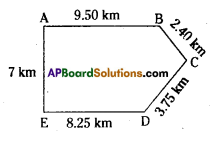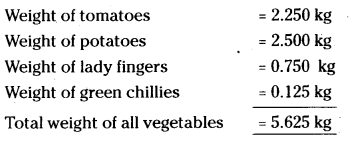# HSSlive: Plus One & Plus Two Notes & Solutions for Kerala State Board

## AP Board Class 7 Maths Chapter 2 Fractions Decimals and Rational Numbers Ex 5 Textbook Solutions PDF: Download Andhra Pradesh Board STD 7th Maths Chapter 2 Fractions Decimals and Rational Numbers Ex 5 Book AnswersAP Board Class 7 Maths Chapter 2 Fractions Decimals and Rational Numbers Ex 5 Textbook Solutions PDF: Download Andhra Pradesh Board STD 7th Maths Chapter 2 Fractions Decimals and Rational Numbers Ex 5 Book Answers

## Andhra Pradesh State Board Class 7th Maths Chapter 2 Fractions Decimals and Rational Numbers Ex 5 Books Solutions

 Board AP Board Materials Textbook Solutions/Guide Format DOC/PDF Class 7th Subject Maths Chapters Maths Chapter 2 Fractions Decimals and Rational Numbers Ex 5 Provider Hsslive

## How to download Andhra Pradesh Board Class 7th Maths Chapter 2 Fractions Decimals and Rational Numbers Ex 5 Textbook Solutions Answers PDF Online?

2. Click on the Andhra Pradesh Board Class 7th Maths Chapter 2 Fractions Decimals and Rational Numbers Ex 5 Answers.
3. Look for your Andhra Pradesh Board STD 7th Maths Chapter 2 Fractions Decimals and Rational Numbers Ex 5 Textbooks PDF.
4. Now download or read the Andhra Pradesh Board Class 7th Maths Chapter 2 Fractions Decimals and Rational Numbers Ex 5 Textbook Solutions for PDF Free.

## AP Board Class 7th Maths Chapter 2 Fractions Decimals and Rational Numbers Ex 5 Textbooks Solutions with Answer PDF Download

Find below the list of all AP Board Class 7th Maths Chapter 2 Fractions Decimals and Rational Numbers Ex 5 Textbook Solutions for PDF’s for you to download and prepare for the upcoming exams:

Question 1.
Which one is greater?
(i) 0.7 or 0.07
(ii) 7 or 8.5
(iii) 1.47or 1.51
(iv) 6 or 0.66
1 cm = 10 mm
1 m = 100cm
1 km = 1000m
1kg =1000gm
Solution:
i) 0.7 or 0.07 = 0.7 is greater
ii) 7 or 8.5= 8.5 is greater
iii) 1.47 or 1.50 = 1.50 is greater
iv) 6 or 0.66 = 6 is greater

Question 2.
Express the following as rupees using decimals.
(i) 9 paise
(ii) 77 rupees 7 paise
(iii) 235 paise
Solution:
(i) 9 paise = 9100 = ₹ 0.09
(ii) 77 rupees 7 paise = 77 rupees 7100 rupees = ₹ 77.07
(iii) 235 paise = ₹ 235100 = ₹ 2.35

Question 3.
(i) Express 10 cm in metre and kilometre.
(ii) Express 45 mm in centimeter, meter and kilometer.
Solution:
i) 10cm = 10100 m = 0.1 m
1o cm = 10100×1000 km = 0.0001 km

ii) 45 mm = 4510 cm = 4.5 cm
= 4.5100 m = 0.045 m
= 0.0451000 km = 0.000045 km

Question 4.
Express the following in kilograms.
(i) 190g
(ii) 247g
(iii) 44kg 80gm
Solution:
(i) 190g = 1901000 = 0.190 kg
(ii) 247g = 2471000 kg = 0.247 kg
(iii) 44kg 80gm = 44 kg 801000 kg = 44.080kg

Question 5.
Write the following decimal numbers in expanded form.
(i) 55.5
(ii) 5.55
(iii) 303.03
(iv) 30.303
(v) 1234.56
Solution:
(i) 55.5 = 10 × 5 + 1 × 5 × 110 × 5 = 50 + 5 + 510
(ii) 5.55 = 1 × 5 + 110 × 5 + 1100 × 5 = 5 + 510+5100
(iii) 303.03 = 100 × 3 + 1 × 3 + 1100 × 3 = 300 + 3 + 3100
(iv) 30.303 = 10 × 3 + 110 x 3 + 11000 x 3 = 30 + 310+31000
(v) 1234.56 = 1000 × 1 + 100 × 2 + 10 × 3 + 1 × 4 + 110 × 5 + 1100 × 6 = 1000 + 200 + 30 + 4 + 510+6100

Question 6.
Write the place value of 3 in the following decimal numbers.
(i) 3.46
(ii) 32.46
(iii) 7.43
(iv) 90.30
(v) 794.037
Solution:
i) 3.46 – place value of 3 in 3.46 is 3 × 1 =-3
ii) 32.46- place value of 3 in 32.46 is 3 × 10 = 30
iii) 7.43- place value of 3 in 7.43 is 3 × 1100 = 0.03
iv) 90.30- place value of 3 in 90.30 is 3 × 110 = 0.3
v) 794.037 – place value of 3 in 794.037 is 3 × 1100 = 0.03

Question 7.
Aruna and Radha start their journey from two different places. A and E. Aruna chose the path from A to B then to km C, while Radha chose the path from E to D then to C. Find who traveled more and by how much?
Solution:
Distance covered by Aruna = AB + BC
= 9.50+2.40 = 11.90km
Distance covered by Rad ha = ED + DC
= 8.25 + 3.75 = 12 km
Radha travelled more by (12.00 – 11.90) = 0.10 km.Question 8.
Upendra went to the market to buy vegetables. He brought 2 kg 250 gm tomatoes, 2 kg 500gm potatoes, 750gm lady fingers and 125 gm green chillies. How much weight did Upendra cany back to his house?Upendra carried back a total weight of 5.625 kg

## Andhra Pradesh Board Class 7th Maths Chapter 2 Fractions Decimals and Rational Numbers Ex 5 Textbooks for Exam Preparations

Andhra Pradesh Board Class 7th Maths Chapter 2 Fractions Decimals and Rational Numbers Ex 5 Textbook Solutions can be of great help in your Andhra Pradesh Board Class 7th Maths Chapter 2 Fractions Decimals and Rational Numbers Ex 5 exam preparation. The AP Board STD 7th Maths Chapter 2 Fractions Decimals and Rational Numbers Ex 5 Textbooks study material, used with the English medium textbooks, can help you complete the entire Class 7th Maths Chapter 2 Fractions Decimals and Rational Numbers Ex 5 Books State Board syllabus with maximum efficiency.

## FAQs Regarding Andhra Pradesh Board Class 7th Maths Chapter 2 Fractions Decimals and Rational Numbers Ex 5 Textbook Solutions

#### Can we get a Andhra Pradesh State Board Book PDF for all Classes?

Yes you can get Andhra Pradesh Board Text Book PDF for all classes using the links provided in the above article.

## Important Terms

Andhra Pradesh Board Class 7th Maths Chapter 2 Fractions Decimals and Rational Numbers Ex 5, AP Board Class 7th Maths Chapter 2 Fractions Decimals and Rational Numbers Ex 5 Textbooks, Andhra Pradesh State Board Class 7th Maths Chapter 2 Fractions Decimals and Rational Numbers Ex 5, Andhra Pradesh State Board Class 7th Maths Chapter 2 Fractions Decimals and Rational Numbers Ex 5 Textbook solutions, AP Board Class 7th Maths Chapter 2 Fractions Decimals and Rational Numbers Ex 5 Textbooks Solutions, Andhra Pradesh Board STD 7th Maths Chapter 2 Fractions Decimals and Rational Numbers Ex 5, AP Board STD 7th Maths Chapter 2 Fractions Decimals and Rational Numbers Ex 5 Textbooks, Andhra Pradesh State Board STD 7th Maths Chapter 2 Fractions Decimals and Rational Numbers Ex 5, Andhra Pradesh State Board STD 7th Maths Chapter 2 Fractions Decimals and Rational Numbers Ex 5 Textbook solutions, AP Board STD 7th Maths Chapter 2 Fractions Decimals and Rational Numbers Ex 5 Textbooks Solutions,
Share: